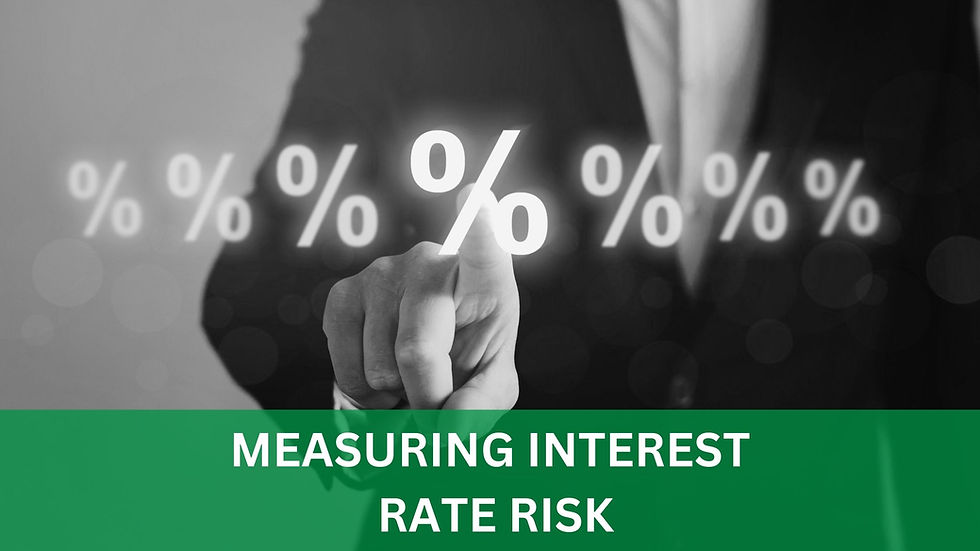top of page

# Measuring Interest Rate Risk

By: John Trefethen, Director & Co-Founder## Measuring Interest Rate Risk

To effectively manage interest rate risk, institutions need timely and accurate information on how changes in interest rates will impact their balance sheet and income statement. This is especially relevant for financial institutions such as credit unions and banks. There are many methods used for measuring exposure to interest rates. Three of the more widely used methods used are 1) gap analysis models, 2) economic value of equity / net economic value models and 3) net interest income simulation models. Below is a description of each.

## Gap Analysis Model

Gap analysis measures the difference between the amount of rate-sensitive assets and rate-sensitive liabilities that collectively will reprice during a specific timeframe in the future. Depending on your view of the direction of future interest rates, the gap analysis will dictate if you should expect positive or negative impact on net income from the rate-sensitive assets and liabilities. The table below summarizes the net income effect for the various assumptions and outcomes of a gap analysis.RSA = rate sensitive assets RSL = rate sensitive liabilities

Gap analysis can help you recognize the timeframe and impact of repricing. However, it has limitations. It cannot measure the effect of embedded options, the effect of non-linear yield shifts, or basis risk. Depending on the complexity of your activities and holdings, it may be necessary to incorporate additional measurement tools.

## Economic Value of Equity (EVE) / Net Economic Value Models (NEV)

EVE and NEV models reflect the net present value of an institution’s assets, liabilities, and off-balance sheet cash flows. EVE and NEV can be used to measure an institution’s long-term interest rate risk by capturing the impact of changes in interest rates in future cash flows through interest rate stress tests. There are limitations in these models. Cash flows can be difficult to quantify for financial instruments that do not have a defined maturity. This creates uncertainty with respect to duration and cash flows. These models are often not effective at valuing embedded options, and generally should not be used to assess more complex instruments. However, these models remain a key interest rate risk tool that allow institutions to prepare for changing interest rate environments.## Net Interest Income Simulation Models

Net interest income simulation models measure the effect that interest rate changes will have on net interest income. If calibrated properly, this model will capture the following four types of interest rate risk:

• Repricing

• Basis

• Yield Curve

• Option

Net interest income is simply interest income minus interest expense. In the model, a baseline of the balance sheet is established. The baseline is the balance sheet’s performance in a static interest rate environment. The balance sheet is then subjected to different interest rate scenarios (stress tests and shock scenarios) and compared to the baseline. A higher variance in these results reflects higher interest rate risk in the balance sheet.## Summary

All three models are capable of showing the potential effects of a changing interest rate environment. But like all models, their effectiveness is limited on the quality of the input assumptions. “Garbage in, garbage out” certainly applies when measuring interest rate risk with any of these models. Managers who review these models should understand the rationale behind assumptions made in the inputs, and changes made to any of the models.

If you would like to speak to someone about interest rate you can email or call John Trefethen:

See All